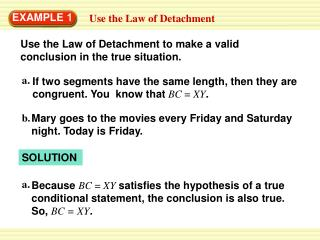# EXAMPLE 1 - PowerPoint PPT PresentationDownload PresentationEXAMPLE 1

EXAMPLE 1
Download Presentation## EXAMPLE 1

- - - - - - - - - - - - - - - - - - - - - - - - - - - E N D - - - - - - - - - - - - - - - - - - - - - - - - - - -
##### Presentation Transcript

1. a. If two segments have the same length, then they are congruent. You know that BC = XY. b. Mary goes to the movies every Friday and Saturday night. Today is Friday. a. Because BC = XYsatisfies the hypothesis of a true conditional statement, the conclusion is also true. So, BC=XY. EXAMPLE 1 Use the Law of Detachment Use the Law of Detachment to make a valid conclusion in the true situation. SOLUTION

2. b. First, identify the hypothesis and the conclusion of the first statement.The hypothesis is “If it is Friday or Saturday night,” and the conclusion is “then Mary goes to the movies.” EXAMPLE 1 Use the Law of Detachment “Today is Friday” satisfies the hypothesis of the conditional statement, so you can conclude that Mary will go to the movies tonight.

3. a. If Rick takes chemistry this year, then Jesse will be Rick’s lab partner.If Jesse is Rick’s lab partner, then Rick will get an A in chemistry. b. Ifx2> 25,thenx2> 20. c. If a polygon is regular, then all angles in the interior of the polygon are congruent. EXAMPLE 2 Use the Law of Syllogism If possible, use the Law of Syllogism to write a new conditional statement that follows from the pair of true statements. Ifx> 5,thenx2> 25. If a polygon is regular, then all of its sides are congruent.

4. a. The conclusion of the first statement is the hypothesis of the second statement, so you can write the following new statement. b. Notice that the conclusion of the second statement is the hypothesis of the first statement, so you can write the following new statement. EXAMPLE 2 Use the Law of Syllogism SOLUTION If Rick takes chemistry this year, then Rick will get an A in chemistry. Ifx > 5,thenx2> 20.

5. c. Neither statement’s conclusion is the same as the other statement’s hypothesis. You cannot use the Law of Syllogism to write a new conditional statement. EXAMPLE 2 Use the Law of Syllogism

6. 1. If 90°< mR < 180°, then Ris obtuse. The measure of Ris 155°. Using the Law of Detachment, what statement can you make? ANSWER Ris obtuse for Examples 1 and 2 GUIDED PRACTICE

7. 2. If Jenelle gets a job, then she can afford a car. If Jenelle can afford a car, then she will drive to school. Using the Law of Syllogism, what statement can you make ? ANSWER Notice that the conclusion of the first statement is the hypothesis of the second statement, So you can write the following statement. If Jenelle gets a job, then she will drive to school. for Examples 1 and 2 GUIDED PRACTICE

8. 3. If you get an A or better on your math test, then you can go to the movies. If you go to the movies, then you can watch your favorite actor. ANSWER Law of Syllogism. for Examples 1 and 2 GUIDED PRACTICE State the law of logic that is illustrated. If you get an A or better on your math test, then you can watch your favorite actor.

9. 4. Ifx > 12, thenx + 9 > 20. The value ofxis 14 Therefore,x + 9 > 20 ANSWER Law of Detachment for Examples 1 and 2 GUIDED PRACTICE